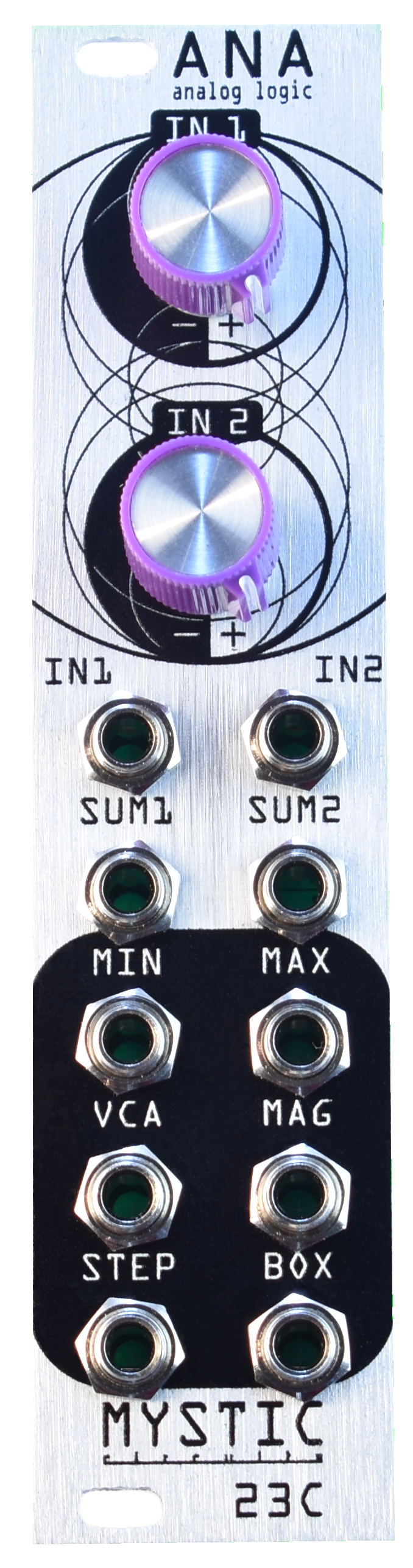\$ 180.00 USD

# Ana

Ana is an analog logic utility that can perform a wide variety of functions. At its core Ana is a set of four diode logic circuits and signals derived from those circuits. However, Ana differs from other logic modules in that it can process variable analog signals and give a variable analog output instead of just binary on-off gates. Use Ana with one input and gain six outputs all related to that input, or feed Ana with two signals and get six cross-modulation processes. With two input controls which work as either attenuverters, offsets or static values Ana can process audio, sequences, modulation and even video in a huge variety of ways.

ANA is a utility module comprising six functions that combine two inputs. These functions are:

MIN – compute the minimum of the two input voltages

MAX – compute the maximum of the two input voltages

VCA – compute the XOR, or multiplication the two input voltages

MAG – compute the difference between the two input voltages.

STEP – perform a track and hold based smooth/ stepped process from the two inputs

BOX – perform a bipolar comparator process resulting in a 3 level square wave.

~

The front panel controls are used to attenuvert or offset the input signals depending on how ANA is patched:

Patching a signal into either IN1 or IN2 will make the corresponding knob function as an attenuverter

Patching a signal into either SUM1 or SUM2 will make the corresponding knob function as an offset

Patching into both inputs will attenuvert the signal patched into either IN1 or IN2 and add the signal patched into either SUM1 or SUM2.

Leaving all inputs to a channel unpatched makes the corresponding knob a static offset.

The attenuverters are able to boost an incoming signal by a factor of 2.

~

ANA lends itself to a wide variety of functions, many of them performed simultaneously.  Here is a short list of them:

Process CV with gain and offset.

Multiply signals as a through-zero VCA with unique character.

Add harmonics by rectifying a signal.

Create pseudo random sequences.

Distort incoming signals under voltage control

Compute Boolean logic on gates or continuous voltages.

Extract Gates and sequences from modulation signals.\$ 180.00 USD

# Ana

Ana is an analog logic utility that can perform a wide variety of functions. At its core Ana is a set of four diode logic circuits and signals derived from those circuits. However, Ana differs from other logic modules in that it can process variable analog signals and give a variable analog output instead of just binary on-off gates. Use Ana with one input and gain six outputs all related to that input, or feed Ana with two signals and get six cross-modulation processes. With two input controls which work as either attenuverters, offsets or static values Ana can process audio, sequences, modulation and even video in a huge variety of ways.

ANA is a utility module comprising six functions that combine two inputs. These functions are:

MIN – compute the minimum of the two input voltages

MAX – compute the maximum of the two input voltages

VCA – compute the XOR, or multiplication the two input voltages

MAG – compute the difference between the two input voltages.

STEP – perform a track and hold based smooth/ stepped process from the two inputs

BOX – perform a bipolar comparator process resulting in a 3 level square wave.

~

The front panel controls are used to attenuvert or offset the input signals depending on how ANA is patched:

Patching a signal into either IN1 or IN2 will make the corresponding knob function as an attenuverter

Patching a signal into either SUM1 or SUM2 will make the corresponding knob function as an offset

Patching into both inputs will attenuvert the signal patched into either IN1 or IN2 and add the signal patched into either SUM1 or SUM2.

Leaving all inputs to a channel unpatched makes the corresponding knob a static offset.

The attenuverters are able to boost an incoming signal by a factor of 2.

~

ANA lends itself to a wide variety of functions, many of them performed simultaneously.  Here is a short list of them:

Process CV with gain and offset.

Multiply signals as a through-zero VCA with unique character.

Add harmonics by rectifying a signal.

Create pseudo random sequences.

Distort incoming signals under voltage control

Compute Boolean logic on gates or continuous voltages.

Extract Gates and sequences from modulation signals.